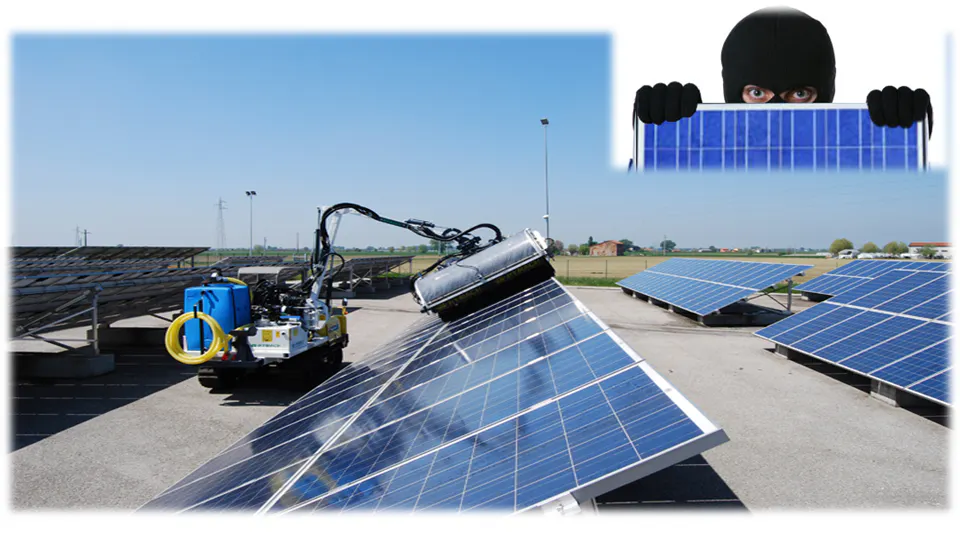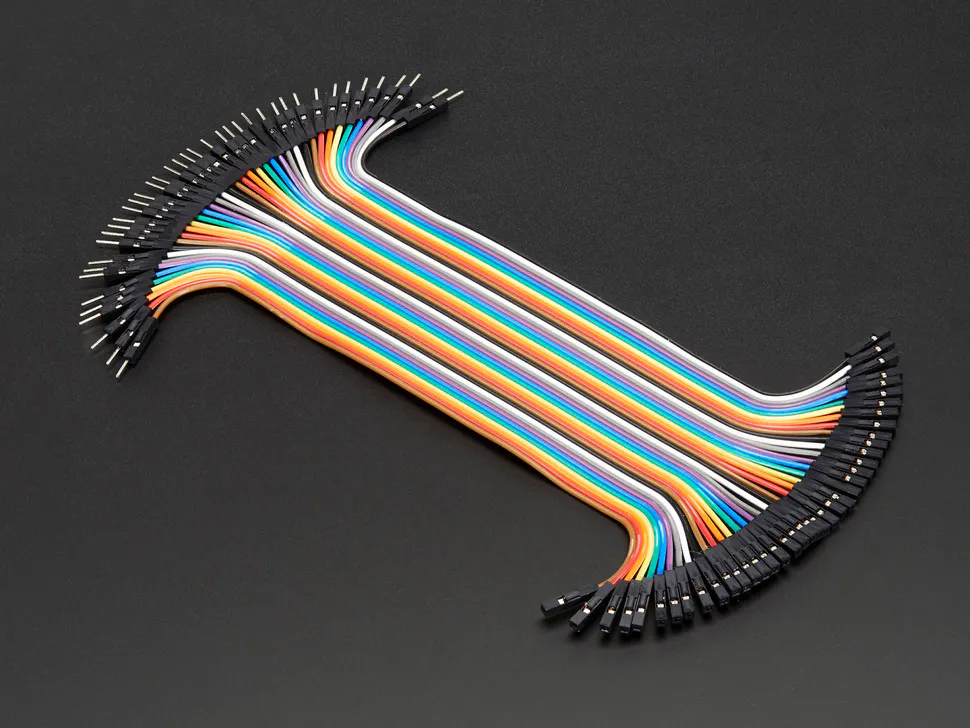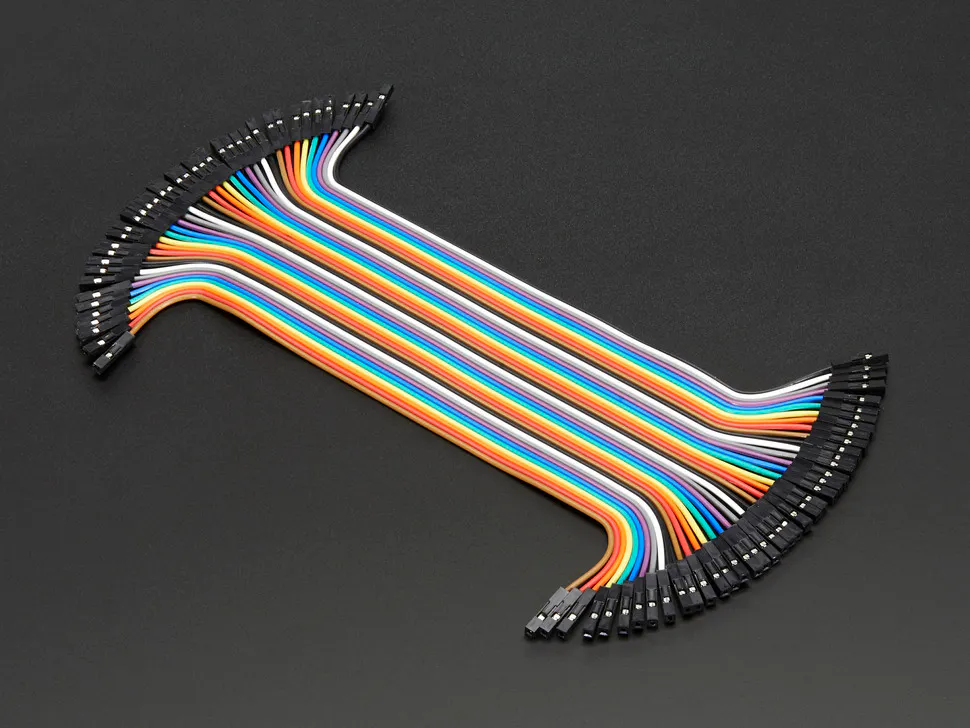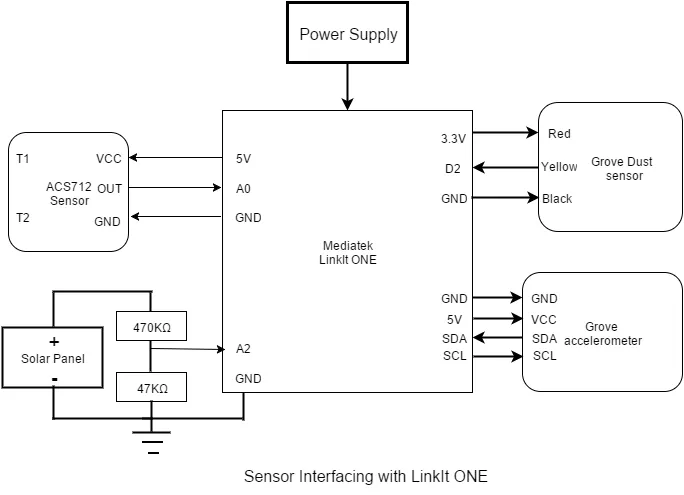Solar Panel Dual Management System (SP DMS)

This IoT prototype performs two functions: 1) Theft prevention and 2) Maintenance Indication of solar panel using LinkIt ONE and Sensors

IntermediateFull instructions provided3 days8,218Things used in this project

Hardware components

 Grove Dust Sensor
×1
 Grove 3-axis accelerometer sensor
×1
 ACS712 current sensor module (5A)
×1
 Resistors 47k
×1
 Resistor 470k
×1
 Male/Male Jumper Wires Components mention after this needed only if you build base shield
×1Male/Female Jumper Wires
×1Female/Female Jumper Wires
×1
 General Purpose PCB
×1
 2.54mm pitch pin headers - Female 40pin in 1 line
×1
 2.54mm pitch 40 pin male header strip
×1
 2pin Screw terminal
×1

Software apps and online servicesArduino IDEUbidots

Hand tools and fabrication machines

 MultimeterSoldering iron (generic) Required only when making base shield
 PCB cutter Required only when making base shield

Schematics

Sensors Interfacing Block DiagramSensors Interfacing Block DiagramCode

Theft_protection_accelerometer.ino

Arduino
#include <Wire.h>      //library to establised I2C communication between LinkIt One and Grove - 3-Axis Digital Accelerometer(16g)
#include <ADXL345.h>  //This library of ADXL345 used extract data read by sensor in various forms

int x_initial,y_initial,z_initial;
double xyz_initial;
double ax_initial,ay_initial,az_initial;

//Function to Store initial axis value of x,y & z for comparision
void axis_initialization(){
adxl.readXYZ(&x_initial, &y_initial, &z_initial); //read the accelerometer values and store them in variables  x_initial,y_initial,z_initial
ax_initial = xyz_initial;
ay_initial = xyz_initial;
az_initial = xyz_initial;
delay(500);
}

//To compare initial values with current reading of x,y & z values for theft protection
int compareResult(){
//Boring accelerometer stuff
int x,y,z,Xchange,Ychange,Zchange;
adxl.readXYZ(&x, &y, &z); //read the accelerometer values and store them in variables  x,y,z
// Output x,y,z values

Serial.print("values of X , Y , Z: ");
Serial.print(x);
Serial.print(" , ");
Serial.print(y);
Serial.print(" , ");
Serial.println(z);

double xyz;
double ax,ay,az;
ax = xyz;
ay = xyz;
az = xyz;

Serial.print("X=");
Serial.print(ax);
Serial.println(" g");
Serial.print("Y=");
Serial.print(ay);
Serial.println(" g");
Serial.print("Z=");
Serial.println(az);
Serial.println(" g");
Serial.println("**********************");

if((int)ax!=(int)ax_initial){
Xchange=1;
}
else {
Xchange=0;
}

Serial.print("Xchange:");
Serial.println(Xchange);

if((int)ay!=(int)ay_initial){
Ychange=1;
}
else {
Ychange=0;
}

Serial.print("Ychange:");
Serial.println(Ychange);

if((int)az!=(int)az_initial){
Zchange=1;
}
else {
Zchange=0;
}

Serial.print("Zchange:");
Serial.println(Zchange);

if((Xchange==1)||(Ychange==1)||(Zchange==1)){
return 1;
}
else return 0;

}
void setup(){
Serial.begin(9600);
delay(5000);
axis_initialization();
Serial.println("SP DMS");
}

void loop(){
int unauthorised_activity;
unauthorised_activity=compareResult();
if(unauthorised_activity==1){
Serial.println("There is unauthorised activity...");
}
delay(500);

}

Grove_dust_sensor.ino

Arduino
/*
Interface to Shinyei Model PPD42NS Particle Sensor
Program by Christopher Nafis
Written April 2012

http://www.seeedstudio.com/depot/grove-dust-sensor-p-1050.html
http://www.sca-shinyei.com/pdf/PPD42NS.pdf

JST Pin 1 (Black Wire)  => Arduino GND
JST Pin 3 (Red wire)    => Arduino 5VDC
JST Pin 4 (Yellow wire) => Arduino Digital Pin 8
*/

int pin = 2;
unsigned long duration;
unsigned long starttime;
unsigned long sampletime_ms = 30000;
unsigned long lowpulseoccupancy = 0;
float ratio = 0;
float concentration = 0;

void setup() {
Serial.begin(9600);
pinMode(2,INPUT);
starttime = millis();
}

void loop() {
duration = pulseIn(pin, LOW);
lowpulseoccupancy = lowpulseoccupancy+duration;

if ((millis()-starttime) > sampletime_ms)
{
ratio = lowpulseoccupancy/(sampletime_ms*10.0);  // Integer percentage 0=>100
concentration = 1.1*pow(ratio,3)-3.8*pow(ratio,2)+520*ratio+0.62; // using spec sheet curve
Serial.print(lowpulseoccupancy);
Serial.print(",");
Serial.print(ratio);
Serial.print(",");
Serial.println(concentration);
lowpulseoccupancy = 0;
starttime = millis();
}
}

Arduino
uint32_t analogPin = A0;
uint32_t value = 0;

float vout = 0.0;
float vin = 0.0;
float R1 = 470000.0;// resistance of R1 (470k)
float R2 = 47000.0; // resistance of R2 (47K)

void setup()
{
Serial.begin(9600);
}
void loop()
{
Serial.print("Analog Value =");
Serial.println(value);
vout = (value * 5.0) / 1024.0;
vin = vout*(R1+R2)/R2;
Serial.print("Voltage =");
Serial.println(vin);
delay(2000);
}

Arduino
uint32_t analogPin = A2;
uint32_t value = 0;

float vout = 0.0;
float vin = 0.0;
float R1 = 470000.0; // resistance of R1 (470k)
float R2 = 47000.0; // resistance of R2 (47K)

void setup()
{
Serial.begin(9600);
}
void loop()
{
float avgVolt=0,clb;
for(int i=0;i<100;i++){
vout = (value * 5.0) / 1024.0;
vin = vout*(R1+R2)/R2;
clb=vin*0.767;
vin=vin+clb;
avgVolt=avgVolt+vin;
}
avgVolt=avgVolt/100.0;
Serial.print("Voltage =");
Serial.println(avgVolt,1);

}

current_sensor_test.ino

Arduino
//Code to measure current AC/DC using ACS712 sensor module(5A model)
// the setup routine runs once when you press reset:
void setup() {
Serial.begin(9600);    // initialize serial communication at 9600 bits per second:
}

// the loop routine runs over and over again forever:
void loop() {
float average = 0;
for(int i = 0; i < 1000; i++) {
average = average + (.0264 * analogRead(A0)-13.51);
delay(1);
}
Serial.print(average);
Serial.println("mA");
}

Arduino
Combine test code for current and voltage.
uint32_t value = 0;

float vout = 0.0;
float vin = 0.0;
float R1 = 470000.0; // resistance of R1 (470k)
float R2 = 47000.0; // resistance of R2 (47K)

// the setup routine runs once when you press reset:
void setup() {
Serial.begin(9600);    // initialize serial communication at 9600 bits per second:
}

// the loop routine runs over and over again forever:
void loop() {
float avgCurrent = 0,avgVolt=0,clb;
for(int i = 0; i < 1000; i++) {
avgCurrent = avgCurrent + (.0264 * analogRead(A0)-13.51);
delay(1);
}
Serial.print("  Current =");
Serial.print(avgCurrent);
Serial.print("mA");
for(int i=0;i<100;i++){
vout = (value * 5.0) / 1024.0;
vin = vout*(R1+R2)/R2;
clb=vin*0.767;
vin=vin+clb;
avgVolt=avgVolt+vin;
}
avgVolt=avgVolt/100.0;
Serial.print("\tVoltage =");
Serial.println(avgVolt,1);
}

Arduino
All sensors combine test code.
#include <Wire.h>      //library to establised I2C communication between LinkIt One and Grove - 3-Axis Digital Accelerometer(16g)
#include <ADXL345.h>  //This library of ADXL345 used extract data read by sensor in various forms

//Accelerometer variables

//variables to store the initial state of device after installation when power on
int x_initial,y_initial,z_initial;
double xyz_initial;
double ax_initial,ay_initial,az_initial;

//variables for Voltage reading
uint32_t Volt_value = 0;
float vout = 0.0;
float vin = 0.0;
float R1 = 470000.0; // resistance of R1 (470k)
float R2 = 47000.0; // resistance of R2 (47K)

//variables for Dust Sensor
int pin = 2;
unsigned long duration;
unsigned long starttime;
unsigned long sampletime_ms = 30000;
unsigned long lowpulseoccupancy = 0;
float ratio = 0;
float concentration = 0;

//Function to Store initial axis value of x,y & z for comparision
void axis_initialization(){
adxl.readXYZ(&x_initial, &y_initial, &z_initial); //read the accelerometer values and store them in variables  x_initial,y_initial,z_initial
ax_initial = xyz_initial;
ay_initial = xyz_initial;
az_initial = xyz_initial;
delay(500);
}

//To compare initial values with current reading of x,y & z values for theft protection
int compareResult(){
//Boring accelerometer stuff
int x,y,z,Xchange,Ychange,Zchange;
adxl.readXYZ(&x, &y, &z); //read the accelerometer values and store them in variables  x,y,z
// Output x,y,z values
double xyz;
double ax,ay,az;
ax = xyz;
ay = xyz;
az = xyz;

if((int)ax!=(int)ax_initial){
Xchange=1;
}
else {
Xchange=0;
}

if((int)ay!=(int)ay_initial){
Ychange=1;
}
else {
Ychange=0;
}

if((int)az!=(int)az_initial){
Zchange=1;
}
else {
Zchange=0;
}

if((Xchange==1)||(Ychange==1)||(Zchange==1)){
return 1;
}
else return 0;
}

void DustCal(){
duration = pulseIn(pin, LOW);
lowpulseoccupancy = lowpulseoccupancy+duration;

if ((millis()-starttime) > sampletime_ms)
{
ratio = lowpulseoccupancy/(sampletime_ms*10.0);  // Integer percentage 0=>100
concentration = 1.1*pow(ratio,3)-3.8*pow(ratio,2)+520*ratio+0.62; // using spec sheet curve
Serial.print("  lowpulseoccupancy: ");
Serial.print(lowpulseoccupancy);
Serial.print(",");
Serial.print("ratio: ");
Serial.print(ratio);
Serial.print(",");
Serial.print("concentration: ");
Serial.println(concentration);
lowpulseoccupancy = 0;
starttime = millis();
}
Serial.println();
}

void setup(){
Serial.begin(9600);
axis_initialization();
pinMode(2,INPUT);
starttime = millis();
}

void loop(){
int unauthorised_activity=0;
unauthorised_activity=compareResult();

if(unauthorised_activity==1){
Serial.println("There is unauthorised activity...");
}
else {
float avgCurrent = 0,avgVolt=0,clb=0;

for(int i = 0; i < 1000; i++) {
avgCurrent = avgCurrent + (.0264 * analogRead(A0)-13.51);
delay(1);
}
Serial.print("  Current =");
Serial.print(avgCurrent);
Serial.print("mA");
for(int i=0;i<100;i++){
vout = (Volt_value * 5.0) / 1024.0;
vin = vout*(R1+R2)/R2;
clb=vin*0.767;
vin=vin+clb;
avgVolt=avgVolt+vin;
}
avgVolt=avgVolt/100.0;
Serial.print("\tVoltage =");
Serial.print(avgVolt,1);
Serial.print("V");
Serial.print("\tPower =");
Serial.print((avgCurrent/1000.0)*avgVolt);
Serial.println("W");

DustCal();

}
delay(500);
}

index.html

HTML
<!DOCTYPE html>
<html>

<title>Solar Panel Dual Management System</title>
<meta charset="utf-8">
<style>
body {
background: #4285F4;
background-size:100%;
}
h1{
color: #ffffff;
}
.container{
color: #ff0000;
}
</style>
<body>
<h1  align="center">Solar Panel Dual Management System</h1>
</div>
<div class="container" align="right">
<a href="location.html"><button type="button">Track Panel</button></a>
</div>
<div class="SensorsData" align="center">
<iframe width="430" height="280" frameborder="0" src="https://app.ubidots.com/ubi/getchart/lfBlDRazzsEpkEs6urLmZtUG008" style="background: transparent"></iframe>&nbsp&nbsp&nbsp&nbsp
<iframe width="430" height="280" frameborder="0" src="https://app.ubidots.com/ubi/getchart/SQHxvj5U7t2IrQC6rROd9HfGNdo" style="background: transparent"></iframe>
<br><br>
<iframe width="430" height="280" frameborder="0" src="https://app.ubidots.com/ubi/getchart/xxw39mZOOQ9xs1skwdAUBx-HAh0" style="background: transparent"></iframe>&nbsp&nbsp&nbsp&nbsp
<iframe width="430" height="280" frameborder="0" src="https://app.ubidots.com/ubi/getchart/mL0s_GCoL-qGQl0-9kZeSp3YLn4" style="background: transparent"></iframe>
<br><br>
<iframe width="430" height="280" frameborder="0" src="https://app.ubidots.com/ubi/getchart/7Cz3vWe5dorePxvA-sich25LJk4" style="background: transparent"></iframe>
</div>

</body>
</html>

location.html

HTML
<!DOCTYPE html>
<html>

<title>Solar Panel Dual Management System</title>
<style>
body {
background: #4285F4;
background-size:100%;
}
h1{
color: #ffffff;
}
h2{
color: #ffffff;
}
</style>
<body>
<h1  align="center">Solar Panel Dual Management System</h1>
</div>
<div class="container" align="left">
<a href="index.html"><button type="button">Back</button></a>
</div>
<div class="SensorsData" align="center">
<iframe width="870" height="480" frameborder="0" src="https://app.ubidots.com/ubi/getchart/Em9WiAc-RENRvLlgtYbR2-9uSYo" style="background: transparent"></iframe>
</div>
<h2  align="center">GPS Location Of Solar Panel</h2>
</body>
</html>

Project_Code.ino

Arduino
Final working project code. For simplicity I have divided major section of codes in various function which will help you to understand the flow of program.
/********************************************************************************************************************************************/
/*  Here LinkIt ONE reads the value of sensors and updates data on Ubidots webserver                                                        */
/*  During normal operation when no theft occurs LinkIt ONE sends the value of curren,voltage,dust sensor and battery value only            */
/*  Once there is unauthorized activity happens then there is change in accelerometer value and sense by LinkIt,                            */
/*  After this LinkIt ONE sends the GPS location and Battery value to server and alert is indicated by email                                */
/********************************************************************************************************************************************/
#include <LGPS.h>         // LinkIt GPS Library
#include <LBattery.h>     // Want to be able to read the battery charge level
#include <LGPRS.h>        //include the base GPRS library
#include <LGPRSClient.h>  //include the ability to Post and Get information using HTTP
#include <Wire.h>         //library to establised I2C communication between LinkIt One and Grove - 3-Axis Digital Accelerometer(16g)
#include <ADXL345.h>      //This library of ADXL345 used extract data read by sensor in various forms

// These are the variables you will want to change based on your IOT data streaming account / provider
#define URL     "things.ubidots.com"
#define TOKEN   "MuIKgAEIYR4AxCeaWEpsPAxKHmopskmGxM1k45mPrgeQSS0pZuKrXXi0zhse"   //replace with your Ubidots token generated in your profile tab
#define voltID  ""       //create a Voltage sensor variable in Ubidots and put its ID here
#define ampsID  ""       //create a Current sensor variable in Ubidots and put its ID here
#define dustID  ""       //create a Dust sensor variable in Ubidots and put its ID here
#define alertID ""       //create a Alert variable in Ubidots and put its ID here
#define batID   ""       //create a Battery variable in Ubidots and put its ID here

// Create instantiations of the GPRS and GPS functions
LGPRSClient globalClient;    // See this support topic from Mediatek - http://labs.mediatek.com/forums/posts/list/75.page
gpsSentenceInfoStruct info;  // instantiate
String Location = "";        //variable to GPS location latitude and longitude in string form

//Create instantiations of the ADXL345 Accelerometer function

//variables to store the initial state of device after installation when power on
int x_initial,y_initial,z_initial;
double xyz_initial;
double ax_initial,ay_initial,az_initial;

//variables for Voltage and current reading
uint32_t Volt_value = 0;
float vout = 0.0;
float vin = 0.0;
float R1 = 470000.0;                   // resistance of R1 (470k)
float R2 = 47000.0;                    // resistance of R2 (47K)
float avgCurrent = 0,avgVolt=0,clb=0;

//variables for Dust Sensor
int pin = 2;
unsigned long duration;
unsigned long starttime;
unsigned long sampletime_ms = 30000;
unsigned long lowpulseoccupancy = 0;
float ratio = 0;
float concentration = 0;

//function to send data of sensors on webserver
String value = String(LBattery.level());  //calculating the level of battery
String payload;                           //Variable to collect all sensor data for data upload on Webserver
String le;                                //length of the payload in characters
if(alert==1){                              //enters when there is theft
LGPS.getData(&info);                   // Get a GPS fix
if (ParseLocation((const char*)info.GPGGA)) {    // This is where we break out needed location information
Serial.print("Location is: ");
Serial.println(Location);                      // This is the format needed by Ubidots
}
if (Location.length()==0) {                      //enters when no GPS location available otherwise execute else case
payload = "[{\"variable\":\"" alertID "\",\"value\":" + String(alert) + "},{\"variable\":\"" batID "\",\"value\":"+ value + "}]";  //Build the JSON packet without GPS info// with GPS info
}
else {
payload = "[{\"variable\":\"" alertID "\",\"value\":" + String(alert) + "},{\"variable\":\"" batID "\",\"value\":"+ value + ", \"context\":"+ Location + "}]"; //Build the JSON packet with GPS info
}

}
else {
payload = "[{\"variable\":\"" ampsID "\",\"value\":"+ String(avgCurrent)+"},{\"variable\":\"" voltID "\",\"value\":" + String(avgVolt) + "},{\"variable\":\"" dustID "\",\"value\":" + String(concentration) + "},{\"variable\":\"" alertID "\",\"value\":" + String(alert) + "},{\"variable\":\"" batID "\",\"value\":"+ value + "}]";
}
le = String(payload.length());      //this is to calcule the length of payload

// if you get a connection, report back via serial:
Serial.print("Connect to ");
Serial.println(URL);
if (globalClient.connect(URL, 80)){
// Build HTTP POST request
Serial.println("connected");
globalClient.print(F("POST /api/v1.6/collections/values/?token="));
globalClient.print(TOKEN);
globalClient.println(F(" HTTP/1.1"));
globalClient.println(F("Content-Type: application/json"));
globalClient.print(F("Content-Length: "));
globalClient.println(le);
globalClient.print(F("Host: "));
globalClient.println(URL);
globalClient.println();
globalClient.println();
globalClient.println((char)26);   //This terminates the JSON SEND with a carriage return
}
else{
// if you didn't get a connection to the server:
Serial.println("connection failed");
}
delay(100);

// if there are incoming bytes available
// from the server, read them and print them:
if (globalClient.available()){
char c = globalClient.read();
Serial.print(c);
}

// if the server's disconnected, stop the globalClient:
if (!globalClient.available() && !globalClient.connected()){
Serial.println();
Serial.println("disconnecting.");
globalClient.stop();
}

}

boolean ParseLocation(const char* GPGGAstr){
// Refer to http://www.gpsinformation.org/dale/nmea.htm#GGA
// Sample data: \$GPGGA,123519,4807.038,N,01131.000,E,1,08,0.9,545.4,M,46.9,M,,*47

char latarray;
char longarray;
int index = 0;
Serial.println(GPGGAstr);
Serial.print("Fix Quality: ");
Serial.println(GPGGAstr);

if (GPGGAstr=='0') {         //  This is the place in the sentence that shows Fix Quality 0 means no fix
Serial.println("No GPS Fix");
Location = "";                 // No fix then no Location string
return 0;
}

String GPSstring = String(GPGGAstr);
for (int i=20; i<=26; i++) {         // We have to jump through some hoops here
latarray[index] = GPGGAstr[i];     // we need to pick out the minutes from the char array
index++;
}

float latdms = atof(latarray);               // and convert them to a float
float lattitude = latdms/60;                 // and convert that to decimal degrees
String lattstring = String(lattitude);       // Then put back into a string
Location = "{\"lat\":";
if(GPGGAstr == 'S') Location = Location + "-";
Location += GPSstring.substring(18,20) + "." + lattstring.substring(2,4);
index = 0;

for (int i=33; i<=38; i++) {           // And do the same thing for longitude
longarray[index] = GPGGAstr[i];      // the good news is that the GPS data is fixed column
index++;
}
float longdms = atof(longarray);       // and convert them to a float
float longitude = longdms/60;          // and convert that to decimal degrees
String longstring = String(longitude); // Then put back into a string
Location += " ,\"lng\":";

if(GPGGAstr == 'W') Location = Location + "-";
if(GPGGAstr == '0') {
Location = Location + GPSstring.substring(31,33) + "." + longstring.substring(2,4) + "}";
}
else {
Location = Location + GPSstring.substring(30,33) + "." + longstring.substring(2,4) + "}";
}
return 1;
}

//Function to Store initial axis value of x,y & z for comparision
void axisInitialization(){
adxl.readXYZ(&x_initial, &y_initial, &z_initial);   //read the accelerometer values and store them in variables  x_initial,y_initial,z_initial
ax_initial = xyz_initial;
ay_initial = xyz_initial;
az_initial = xyz_initial;
delay(500);
}

//To compare initial values with current reading of x,y & z axis values for theft protection
int compareResult(){
//Boring accelerometer stuff
int x,y,z,Xchange,Ychange,Zchange;
adxl.readXYZ(&x, &y, &z);         //read the accelerometer values and store them in variables  x,y,z
// Output x,y,z values
double xyz;
double ax,ay,az;
ax = xyz;
ay = xyz;
az = xyz;

if((int)ax!=(int)ax_initial){
Xchange=1;
}
else {
Xchange=0;
}

if((int)ay!=(int)ay_initial){
Ychange=1;
}
else {
Ychange=0;
}

if((int)az!=(int)az_initial){
Zchange=1;
}
else {
Zchange=0;
}

if((Xchange==1)||(Ychange==1)||(Zchange==1)){
return 1;
}
else return 0;
}

//Function to calculate voltage, current and power of Solar Panel for Efficieny test
void powerCal(){
avgCurrent = 0,avgVolt=0,clb=0;
for(int i = 0; i < 1000; i++) {
avgCurrent = avgCurrent + (.0264 * analogRead(A0)-13.51);
delay(1);
}
Serial.print("  Current =");
Serial.print(avgCurrent);
Serial.print("mA");
for(int i=0;i<100;i++){
vout = (Volt_value * 5.0) / 1024.0;
vin = vout*(R1+R2)/R2;
clb=vin*0.767;
vin=vin+clb;
avgVolt=avgVolt+vin;
}
avgVolt=avgVolt/100.0;
Serial.print("\tVoltage =");
Serial.print(avgVolt,1);
Serial.print("V");
Serial.print("\tPower =");
Serial.print((avgCurrent/1000.0)*avgVolt);                        //Computing power
Serial.println("W");
}

//Function for calculating the dust concentration level
void dustCal(){
duration = pulseIn(pin, LOW);                                       //reads the duration or total of low pulse on pin
lowpulseoccupancy = lowpulseoccupancy+duration;

if ((millis()-starttime) > sampletime_ms)
{
ratio = lowpulseoccupancy/(sampletime_ms*10.0);                   // Integer percentage 0=>100
concentration = 1.1*pow(ratio,3)-3.8*pow(ratio,2)+520*ratio+0.62; // using spec sheet curve
Serial.print("  lowpulseoccupancy: ");
Serial.print(lowpulseoccupancy);
Serial.print(",");
Serial.print("ratio: ");
Serial.print(ratio);
Serial.print(",");
Serial.print("concentration: ");
Serial.println(concentration);
lowpulseoccupancy = 0;
starttime = millis();
}
Serial.println();
}

//runs only once when power on or reset
void setup(){
Serial.begin(9600);                                  // setup Serial port

LGPS.powerOn();                                      // Start the GPS first as it takes time to get a fix
Serial.println("GPS Powered on, and waiting ...");
Serial.println("Attach to GPRS network");            // Attach to GPRS network - need to add timeout
while (!LGPRS.attachGPRS("","","")) {                //attachGPRS(const char *apn, const char *username, const char *password);
delay(500);
}
LGPRSClient client;                                  //Client has to be initiated after GPRS is established with the correct APN settings - see above link
globalClient = client;                               // Again this is a temporary solution described in support forums
delay(1000);
deviceLocation(0);                                   //Sending device location first time during installation and passing 0 means no theft

delay(3000);                                         //setup time so when power on no error in storing initial axis value of panel
axisInitialization();                                //calling function to store the intial installed axis of system

pinMode(2,INPUT);                                    //Initializing digital pin 2 as input for reading dust sensor value
starttime = millis();                                //Returns the number of milliseconds since the LinIt ONE board began running the current program
}

//forever loop code
void loop(){
int unauth_activity=0;
unauth_activity= compareResult();                         //Function to check or compare the axis value of panel for any change

if(unauth_activity==1){
Serial.println("There is unauthorised activity...");
deviceLocation_data(1);                               //Function for sending device location with battery level for tracing and passing 1, which is alert indication of theft
delay(100);
}
else {
powerCal();               //calling function for calculating voltage, current and power provided by solar panel
dustCal();                //calling function for calculating deposition of dust concentration on panel
deviceLocation_data(0);   //Function for sending sensor data for Maintenance Indication or monitoring of Solar panel for Efficiency check and passing 0 means normal acitvity
}
delay(500);
}

Credits

Brijesh Singh

20 projects • 23 followers
Utilizing the spare time to Make and Share DIY Electronics and IoT projects with the online maker community.